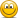# Chapter 5 question 5.5.

#### FRM_STUDY

##### New Member
I am looking at question 5.5: The riskless rate of interes is r=5% and the market portfolio is characterized by E(Rm) = 13% and SD=15%. CAMP implies that the expected return of stocks A, B and C are:

The answer is E(Ra) = -3%, b= 5% and c=21%

A and B I get using = E(Ri)= r +Beta i (E(Rm)-5)
a = 5 +-1(13-5) = -3%
b= 5 +0(13-5) = 5%
c = 5+1(13-5) = 13%

#### David Harper CFA FRM

##### David Harper CFA FRM
Staff member
Subscriber
Hi @FRM_STUDY GARP had MANY of errors in the 2020 print, and that was one of them. Given betas of -1, 0 and 1, your solution WOULD correct! However, I don't think there was even a basis for determining those betas in the prior question. But for some unexplained reason they assumed betas of -1, 0, and 2, so they got the following ...
• a = 5 +-1(13-5) = -3%
• b= 5 +0(13-5) = 5%
• c = 5+2(13-5) = 21%
... then they fixed this mess in the 2021 book where they gave betas of 0.50, 1.0 and 2.0 such that the answer is
• a = 5 +0.5(13-5) = 9%
• b= 5 +1.0(13-5) = 13%
• c = 5+2.0(13-5) = 21%
... so your understand this pattern correctly!#### FRM_STUDY

##### New Member
Hi @FRM_STUDY GARP had MANY of errors in the 2020 print, and that was one of them. Given betas of -1, 0 and 1, your solution WOULD correct! However, I don't think there was even a basis for determining those betas in the prior question. But for some unexplained reason they assumed betas of -1, 0, and 2, so they got the following ...
• a = 5 +-1(13-5) = -3%
• b= 5 +0(13-5) = 5%
• c = 5+2(13-5) = 21%
... then they fixed this mess in the 2021 book where they gave betas of 0.50, 1.0 and 2.0 such that the answer is
• a = 5 +0.5(13-5) = 9%
• b= 5 +1.0(13-5) = 13%
• c = 5+2.0(13-5) = 21%
... so your understand this pattern correctly!Thanks so much for your response, it helps a lot in getting convidence with the formulas!